# Dynamic Arrays

Each new version of Excel includes a variety of minor updates that are nice, but not earth shattering. They can be helpful, but don’t necessarily have a big impact on our workbooks. Indeed, if you ignore them, you can continue using Excel just like before and you’ll probably be just fine.

However, we occasionally receive updates that can dramatically impact our Excel workbooks and are too important to ignore. For example, some of the major enhancements we’ve received over the years are Tables, SUMIFS, Power Query and Power Pivot.

Well, friends, the time is upon us for another major update. Microsoft has been hard at work updating Excel’s calculation engine. Depending on the version of Excel you’re using, you either have — or will have when you update — access to some revolutionary new capabilities that are sure to change how you think about and use Excel.

Let’s dig into a new feature called dynamic arrays. A friendly way to think about it is this: Formulas can return multiple values and when they do, the results automatically spill into the adjacent cells. Let’s back up and unpack that statement.

## Explainer

As we’ve used Excel over the years, we’ve grown accustomed to thinking about formulas as returning a single value, right? You write a formula into a cell and press Enter. The formula does its thing and returns a result. A single result. And that result is displayed in the cell, right? Right. (Technically, they’ve been able to return more than one value, but in general, that is the way we think about formulas.)

Now, try to imagine that a single formula can return many values (aka, an “array”). Go ahead … close your eyes and try to imagine that. You write a formula and hit Enter. The formula returns many values. When you open your eyes, many questions come flooding into your mind. So, let’s take these likely questions one at a time.

## Likely Questions

If I write a formula in a single cell that returns many values, how will they be displayed? Will only the first value be displayed in the formula cell? Will the formula add up all the values and display the sum?

Well, here’s how that works with the new calc engine. The results “spill” out of that formula cell and into the adjacent cells (e.g., the cells below or right of the formula cell) as needed. Don’t panic. If there are any values in those cells, the formula won’t delete them, you’ll get an alert.

But, set that aside for a moment and visualize this: You write a formula and hit Enter. The formula computes and returns many values. These values are all displayed in the worksheet because the results spill out of the formula cell into the cells nearby. The techie term for this area is the “spill range.”

What kind of formulas return multiple values? The functions I use like SUM, COUNT and VLOOKUP all return one value.

There are several new functions that have been introduced initially — with more to come over time — including FILTER, UNIQUE and SORT. These functions can return multiple values and, when they do, the results are returned to the spill range.

When the worksheet is recalculated, what happens if the formula returns more or fewer results than before?

Excel will adjust the spill range automatically based on the number of results, either expanding or contracting as needed. This is a dynamic array because the number of results can change and thus the number of cells in the spill range.

Who cares? How will this improve my workbooks?

This is such a fundamental change to the core Excel calc engine, it will be interesting to see how millions of users will apply dynamic array functions to their workbooks; but, here are a few examples to get you started on this new adventure.

## Examples

### UNIQUE: Create a De-duped Column with a Formula

Let’s say we have several transactions stored in a table named Table1 and perhaps it looks something like Figure 1.Figure 1

Our goal is to obtain a list of the accounts used. One option is to copy/paste the account column and then use the Remove Duplicates command. If this were a one-time project, that’d be just fine. However, when this is a recurring process, we like to eliminate such manual steps.

A new option available to us is to use a dynamic array formula. The UNIQUE function accepts a range, and then returns a list of unique items (it removes duplicates). So, in another area of the worksheet, we use the UNIQUE function in a cell, such as cell F7, like this:

`=UNIQUE(Table1[Account])`

It doesn’t return a single value, it returns an array of multiple values (Travel, Meals, Phone) —and the results are returned into the spill range, like in Figure 2.Figure 2

Now, the good news is that this is a dynamic array. Meaning, next period, when you add new transactions, any new accounts will be added to the account list automatically.

### SORT: Sort That Column

Currently, the results are unsorted—they just appear in the order they’re found in the data source. We can easily sort these results if desired. The SORT function does just that. So, we can easily add the SORT function to our formula, like this:

`=SORT(UNIQUE(Table1[Account]))`

Now, the results are updated accordingly, as seen in Figure 3.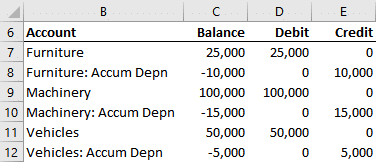Figure 3

Again, as the data table is updated the dynamic array is updated accordingly.

### Spill Reference #: Sum the Values

Let’s now say that we’d like to compute the sum of each of these accounts. We can use the SUMIFS function for that. Since our dynamic array formula was written into F7, we write the following SUMIFS formula into G7:

`=SUMIFS(Table1[Amount],Table1[Account],F7)`

When we hit Enter, we confirm it worked for the first account. See Figure 4.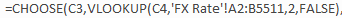Figure 4

However, it didn’t fill itself down. We could fill it down manually, but there’s an easier way. We can use the spill range operator (#) to reference the spill range. So, we update our formula like this:

`=SUMIFS(Table1[Amount],Table1[Account],F7#)`

And now it is filled down automatically, as in Figure 5.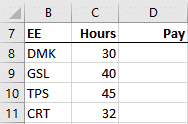Figure 5

Note: SUMIFS has been around for more than a decade, so chances are you are familiar with it; but if not, feel free to check out the SUMIFS blog post referenced below.

### FILTER: Retrieve One Type of Transaction from a Table

Here’s another example. Let’s say we paste a bunch of transactions into a table each month. We’d love it if Excel could somehow create a list of a certain type of transaction on another sheet, regardless of the order they appear in the source table. Historically, this has been accomplished a few different ways. For example, we could apply a filter on the table and the copy/paste. Another option is via PivotTable.

Now there is a new option. Let’s say we paste a bunch of transactions from our accounting system for the sales account. It shows both sales and refunds in a table named Table2, as in Figure 6.Figure 6

Let’s say we want to create a list of the Refund transactions on another sheet. We can use the FILTER function to accomplish this. For example, in a cell we write the following formula:

`=FILTER(Table2,Table2[Type]="Refund")`

That single formula spills down and right, and the results are shown in Figure 7.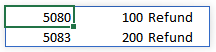Figure 7

### XLOOKUP: Return Multiple Lookup Values

XLOOKUP is a new lookup function that effectively replaces VLOOKUP. In case you aren’t familiar with XLOOKUP, check out my previous CalCPA article about it linked below. It can return multiple values and the results spill into the adjacent cells. For example, we have a range of data stored in a table named Table3, like Figure 8.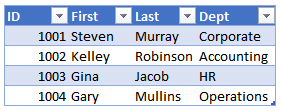Figure 8

We want to return all values for employee ID 1002, so we write this formula:
=XLOOKUP(1002,Table3[ID],Table3)

And it returns all results and spills to the right, as in Figure 9.Figure 9

Note: In practice, we’d probably place the lookup value in a cell and reference the cell location instead.

As you can see, dynamic array functions and spill ranges offer new options, and it will be interesting to see how Excel users will ultimately apply them to their workbooks. And remember, Excel rules!